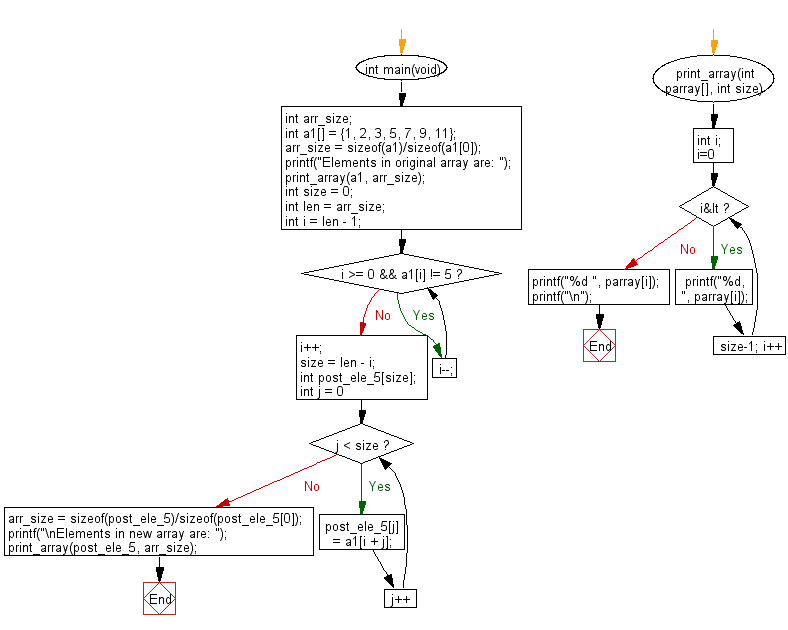﻿ C : Elements after the value 5 from an array of integers# C Exercises: Create a new array taking the elements after the element value 5 from a given array of integers

## C-programming basic algorithm: Exercise-70 with Solution

Write a C program to create a new array taking the elements after the element value 5 from a given array of integers.

C Code:

``````#include <stdio.h>
#include <stdlib.h>
int main(void){
int arr_size;
int a1[] = {1, 2, 3, 5, 7, 9, 11};
arr_size = sizeof(a1)/sizeof(a1);
printf("Elements in original array are: ");
print_array(a1, arr_size);

int size = 0;
int len = arr_size;
int i = len - 1;
while (i >= 0 && a1[i] != 5) i--;
i++;
size = len - i;
int post_ele_5[size];
for (int j = 0; j < size; j++)
{
post_ele_5[j] = a1[i + j];
}
arr_size = sizeof(post_ele_5)/sizeof(post_ele_5);
printf("\nElements in new array are: ");
print_array(post_ele_5, arr_size);
}
print_array(int parray[], int size)
{
int i;
for( i=0; i<size-1; i++)
{
printf("%d, ", parray[i]);
}
printf("%d ", parray[i]);
printf("\n");
}
``````

Sample Output:

```Elements in original array are: 1, 2, 3, 5, 7, 9, 11

Elements in new array are: 7, 9, 11
```

Pictorial Presentation:Flowchart:C Programming Code Editor:

What is the difficulty level of this exercise?

Test your Programming skills with w3resource's quiz.

﻿

## C Programming: Tips of the Day

Why doesn't a+++++b work?

printf("%d",a+++++b); is interpreted as (a++)++ + b according to the Maximal Munch Rule!.

++ (postfix) doesn't evaluate to an lvalue but it requires its operand to be an lvalue.

! 6.4/4 says the next preprocessing token is the longest sequence of characters that could constitute a preprocessing token"

Ref : https://bit.ly/3fdldUT